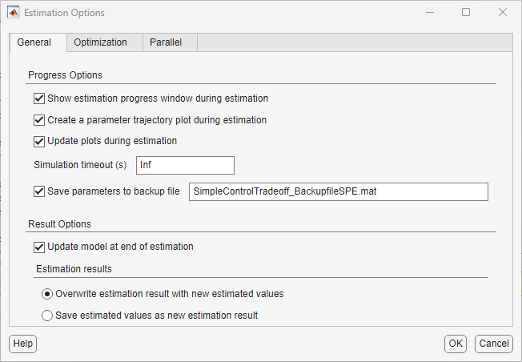## Specify Estimation Options

This topic shows how to specify estimation options in the Parameter Estimator.

After you have specified estimation data and parameters, specify the following estimation options:

1. Goodness of fit criteria (cost function)The cost function is a function that estimation methods minimize. To specify the method for calculating the cost function, in the Parameter Estimation tab of the app, select one of the following from the Cost Function drop-down list:

• `Sum Squared Error` — Uses a least-squares approach (default).

• `Sum-Absolute Error` — Uses the sum of absolute errors.

If the experimental data has many outliers, you can select the robust cost option. The software uses a Huber loss function to handle the outliers in the cost function and improves the fit quality. This option reduces the influence of outliers on the estimation without you having to manually modify your data. To select this option, in the Parameter Estimation tab, clickto open the Estimation Options dialog box. In the Optimization Options tab of the dialog box, select Use robust cost.

The software uses the error statistics to identify the outliers. The error, e, is calculated as the difference between measured and simulated output. The cost function used, F(x), depends on the method used.

Method NameCost Function for NonoutliersCost Function for Outliers
`Sum Squared Error`

`$F\left(x\right)=\sum _{t\in NOL}e\left(t\right)×e\left(t\right)$`

NOL is the set of nonoutlier samples.

`$F\left(x\right)=\sum _{t\in OL}w×|e\left(t\right)|$`

w is a linear weight. OL is the set of outlier samples.

`Sum-Absolute Error`

`$F\left(x\right)=\sum _{t\in NOL}|e\left(t\right)|$`

NOL is the set of nonoutlier samples.

`$F\left(x\right)=\sum _{t\in OL}w$`

w is a constant value. OL is the set of outlier samples.

2. Estimation progress and result options for estimation task

To specify these options, in the Parameter Estimation tab, click to open the Estimation Options dialog box. In the General Options tab, specify the estimation progress and result options. For details about the options, click the Help button.3. Optimization options, such as optimization method and optimization termination options.

Specify these options in the Optimization Options tab of the Estimation Options dialog box. For details about the options, click the Help button.

4. Parallel computing options

Specify these options in the Parallel Options tab of the Estimation Options dialog box. For details about the options, see Estimate Parameters Using Parallel Computing in the Parameter Estimator App.

## Related Examples